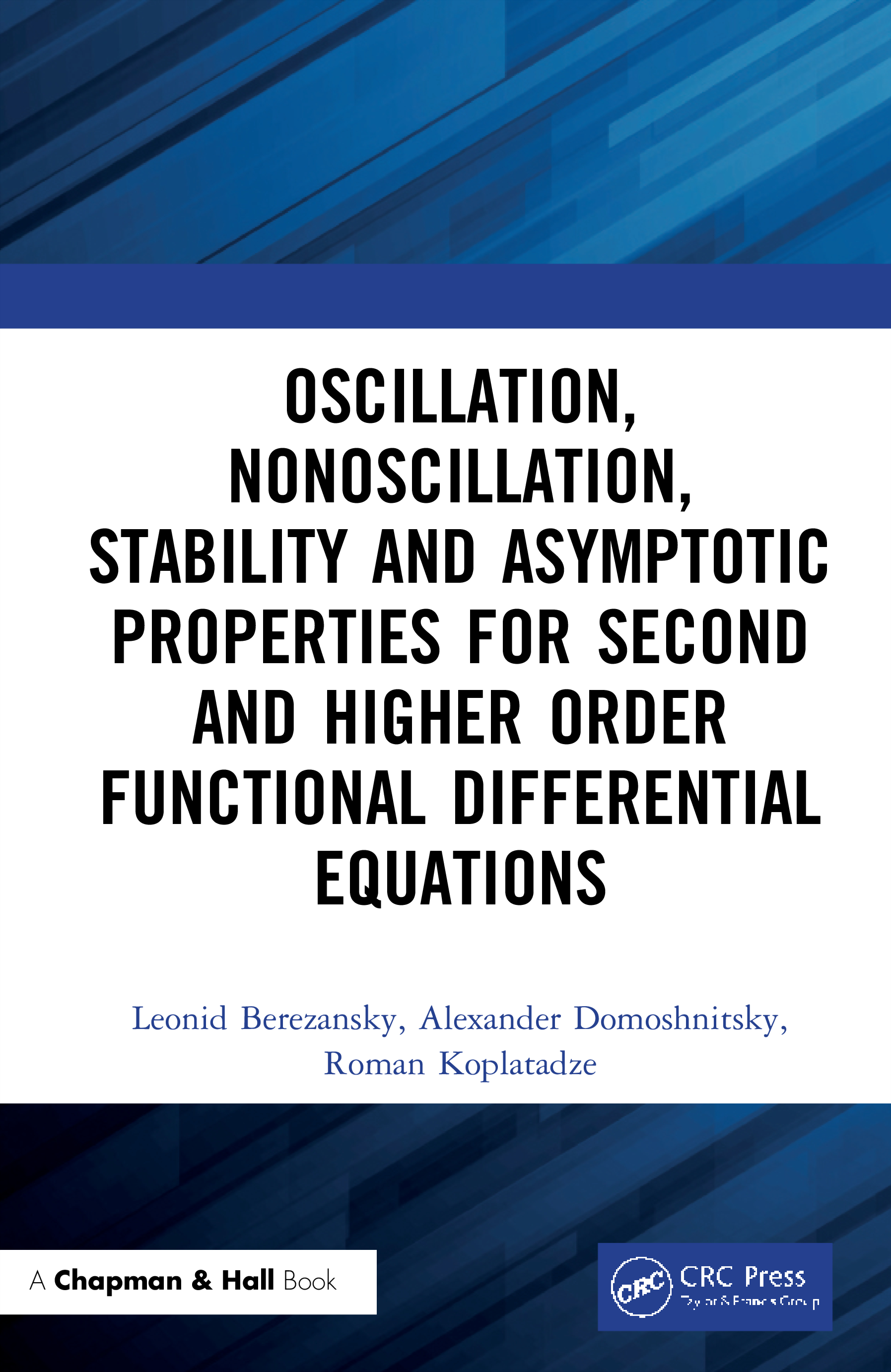# Oscillation, Nonoscillation, Stability and Asymptotic Properties for Second and Higher Order Functional Differential Equations

## 1st Edition

Chapman and Hall/CRC

624 pages

Hardback: 9780367337544
pub: 2020-05-14
SAVE ~\$44.00
Available for pre-order. Item will ship after 14th May 2020
\$220.00
\$176.00
x

FREE Standard Shipping!

### Description

Asymptotic properties of solutions such as stability/ instability,oscillation/ nonoscillation, existence of solutions with specific asymptotic, maximum principles present a classical part in the theory of higher order functional differential equations. The use of these equations in applications is one of the main reasons for the developments in this field. The control in the mechanical processes leads to mathematical models with second order delay differential equations. Stability and stabilization of second order delay equations are one of the main goals of this book. The book is based on the authors’ results in the last decade.

Features:

• Stability, oscillatory and asymptotic properties of solutions are studied in correlation with each other.
• The first systematic description of stability methods based on Bohl-Perron theorem.
• Simple and explicit exponential stability tests.

In this book, various types of functional differential equations are considered: second and higher orders delay differential equations with measurable coefficients and delays, integro-differential equations, neutral equations, operator equations. Oscillation/nonoscillation, existence of unbounded solutions, instability, special asymptotic behavior, positivity, exponential stability and stabilization of functional differential equations are studied. New methods for the study of exponential stability are proposed. Note among them such as the W-transform (right regularization), a priory estimation of solutions, maximum principles, differential and integral inequalities, matrix inequality method, reduction to a system of equations.

The book can be used by applied mathematicians and as a basis for a course on stability of functional differential equations for graduate students.

A Brief Biography. Preface. Introduction to Stability Methods. Stability: A priory Estimation Method. Stability: Reduction to a System of Equations. Stability: W-transform Method I. Stability: W-transform Method II. Exponential Stability for Equations with Positive and Negative Coeffcients. Connection Between Nonoscillation and Stability. Stabilization for Second Order Delay Models, Simple Delay Control. Stabilization by Delay Distributed Feedback Control. Wronskian of Neutral FDE and Sturm Separation Theorem. Vallee-Poussin Theorem for Delay and Neutral DE. Sturm Theorems and Distance between Adjacent Zeros. Unbounded Solutions and Instability of Second Order DDE. Upper and Lower Estimates of Distances Between Zeros and Floquet Theory for Second Order DDE. Distribution of Zeros and Unboundedness of Solutions to Partial DDE. Second Order Equations: Oscillation and Boundary Value Problems. Stability of Third Order DDE. Operator Differential Equations. Properties A and B of Equations with a Linear Minorant. On Kneser-Type Solutions. Monotonically Increasing Solutions. Specific Properties of FDE. A Useful Theorems from Analysis. B Functional-differential Equations. Bibliography. Index.

Leonid Berezansky studied mathematics at Novosibirsk State University, USSR. In 1980, he received Ph.D. degree on Mathematics, at the Institute of Mathematics and Mechanics of the Academy of Sciences of Kazakhstan, Alma-Ata and, in 1991, a Doctor of Science degree at the Institute of Mathematics Academy of Science of USSR, Sverdlovsk with the thesis, Existence of Solutions and Stability of Linear Functional Di erential Equations. In 1991- 1992 he was a full-professor at the Novosibirsk Water Transport Institute, USSR. In 1993 he got a professor tenure (Kameya Programm) at Ben-Gurion University of the Negev, now retired. He is the author of one monograph and more then 150 papers on mathematics and an associate editor in several scientific journals.

Alexander Domoshnitsky studied mathematics at Perm State University, USSR. He received his Ph.D. degree on Mathematics in 1984 in Tbilisi State University, USSR (now Georgia). In 1984-1990, he was on positions of

Senior Lecturer and Dozent (associated Professor) of Perm Polytechnical Institute, Perm, USSR. In 1998-2009, he was Senior Lecturer and Associated Professor of Department of Mathematics and Computer Sciences, Ariel University Center of Samaria, Ariel, Israel. Since 2009 he is Full Professor of Department of Mathematics, Ariel University, Ariel, Israel. He is the author of one monograph and more that 120 papers om mathematics. He is associated editor in several scientific journals.

Roman Koplatadze studied at Tbilisi State University, USSR (now Georgia). He received his Ph.D. degree on Mathematics in 1974 in Tbilisi State University, Georgia, and Doctor of Sciences in 1995 A. Razmadze Mathematical Institute of Georgian Academy of Sciences. In 1970 - 1976 he was on position of junior Researcher of the Department of Ordinary Di erential equations of I. Vekua Institute of Applied Mathematics of Tbilisi State University; 1976-1983 Senior Researcher of the same department; 1983-1990 Leading Researcher of the same department; 1991-1995 Head of the same department; 1996-2006 Leading Researcher of A. Razmadze Mathematical Institute of Georgian Academy of Sciences, Professor; since 2006 present I. Vekua Institute of Applied Mathematics of Iv, Javakhishvili Tbilisi State University, Leading of the same department and Professor at Department of Mathematics of Iv, Javakhishvili Tbilisi State University He is the author of two monographs and more that 130 papers on mathematics. He is deputy of editor of journal Seminar of Ilia Vekua Institute of Applied mathematicsa.